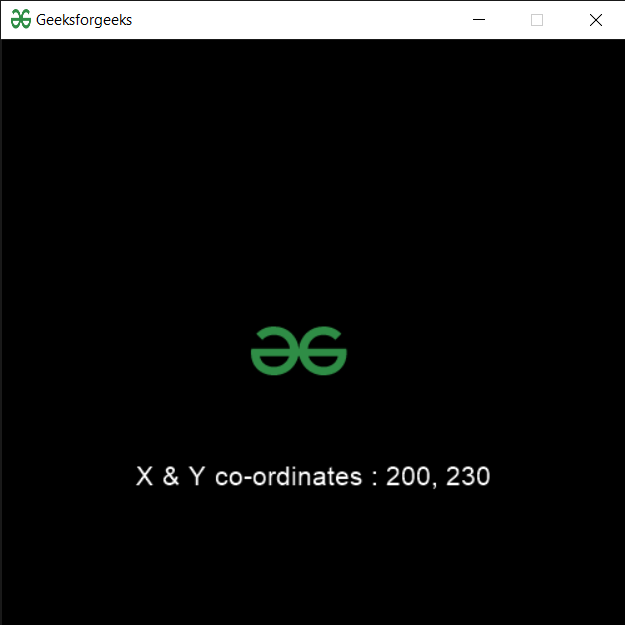# PYGLET – Sprite X and Y Co-ordinates

• Last Updated : 06 Aug, 2021

In this article we will see how we can access X & Y co-ordinates of the sprite in PYGLET module in python. Pyglet is easy to use but powerful library for developing visually rich GUI applications like games, multimedia etc. A window is a “heavyweight” object occupying operating system resources. Windows may appear as floating regions or can be set to fill an entire screen (fullscreen). A sprite is an instance of an image displayed on-screen. Multiple sprites can display the same image at different positions on the screen. Sprites can also be scaled larger or smaller, rotated at any angle and drawn at a fractional opacity. Image is loaded with the help of image module of pyglet. Sprite X & Y co-ordinates are the position of sprite from the x-axis and y-axis.
We can create a window and sprite object with the help of commands given below

```# creating a window
window = pyglet.window.Window(width, height, title)

# creating a sprite object
sprite = pyglet.sprite.Sprite(img, x, y)```

In order to create window we use x and y attribute with sprite object
Syntax : sprite.x and sprite.y
Argument : It takes no argument
Return : It returns integer

Below is the implementation

## Python3

 `# importing pyglet module  ` `import` `pyglet  ` `import` `pyglet.window.key as key ` `   `  `# width of window  ` `width ``=` `500` `   `  `# height of window  ` `height ``=` `500` `   `  `# caption i.e title of the window  ` `title ``=` `"Geeksforgeeks"` `   `  `# creating a window  ` `window ``=` `pyglet.window.Window(width, height, title)  ` `   `  `# text   ` `text ``=` `"Welcome to GeeksforGeeks"` `  `  `# creating label with following properties ` `# font = cooper ` `# position = 250, 150 ` `# anchor position = center ` `label ``=` `pyglet.text.Label(text,  ` `                          ``font_name ``=``'Cooper'``,  ` `                          ``font_size ``=` `16``,  ` `                          ``x ``=` `250``,   ` `                          ``y ``=` `150``,  ` `                          ``anchor_x ``=``'center'``,   ` `                          ``anchor_y ``=``'center'``) ` ` `  ` `  `# creating a batch  ` `batch ``=` `pyglet.graphics.Batch() ` ` `  `# loading geeksforgeeks image ` `image ``=` `pyglet.image.load(``'gfg.png'``) ` ` `  ` `  ` `  `# creating sprite object ` `# it is instance of an image displayed on-screen ` `sprite ``=` `pyglet.sprite.Sprite(image, x ``=` `200``, y ``=` `230``) ` `   `  `# on draw event  ` `@window``.event  ` `def` `on_draw():  ` `       `  `    ``# clear the window  ` `    ``window.clear()  ` `       `  `    ``# draw the label ` `    ``label.draw()  ` `     `  `    ``# draw the image on screen ` `    ``sprite.draw() ` `       `  `# key press event      ` `@window``.event  ` `def` `on_key_press(symbol, modifier):  ` `   `  `    ``# key "C" get press  ` `    ``if` `symbol ``=``=` `key.C:  ` `         `  `        ``# printing the message ` `        ``print``(``"Key : C is pressed"``) ` `         `  `# image for icon  ` `img ``=` `image ``=` `pyglet.resource.image(``"gfg.png"``)  ` ` `  `# setting image as icon  ` `window.set_icon(img)  ` ` `  `# accessing x, y co-ordinates of the sprite ` `value_x ``=` `sprite.x ` `value_y ``=` `sprite.y ` ` `  `# creating text from value ` `text ``=` `"X & Y co-ordinates : "` `+` `str``(value_x) ``+` `", "` `+` `str``(value_y) ` ` `  `# setting text to the label ` `label.text ``=` `text ` `    `  `# start running the application  ` `pyglet.app.run()`

Output :My Personal Notes arrow_drop_up
Recommended Articles
Page :